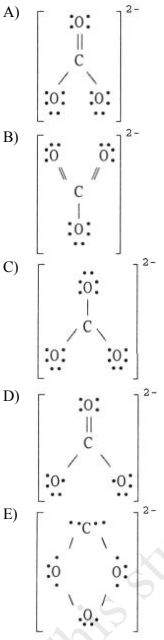# Problem: The Lewis structure of the CO32- ion is __________.

###### FREE Expert Solution

We are being asked to identify the correct structure for CO32-. We have to follow the steps:

Step 1: Determine the central atom in this molecule.

Step 2: Calculate the total number of valence electrons present.

Step 3: Draw the Lewis Structure for the molecule

Step 4: Calculate for formal charges

Step 1: Determine the central atom in this molecule.

C → less electronegative atom than O → central atom

Step 2: Calculate the total number of valence electrons present.

CO32-:

Group              Valence Electrons

C         4A                   1 x 4 e‑  = 4 e‑
O         6A                   3 x 6 e- = 18 e‑
-2 charge                                 2 e‑
Total = 24 valence e-

Step 3: Draw the Lewis Structure for the molecule

88% (475 ratings)###### Problem Details

The Lewis structure of the CO32- ion is __________.